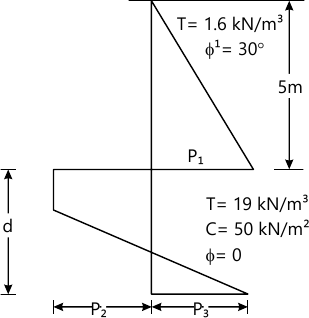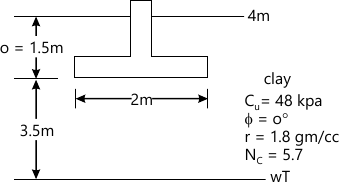MORE IN Geotechnical Engineering - 2
MU Civil Engineering (Semester 6)
Geotechnical Engineering - 2
December 2014
Total marks: --
Total time: --
INSTRUCTIONS
(1) Assume appropriate data and state your reasons
(2) Marks are given to the right of every question
(3) Draw neat diagrams wherever necessary

Attempt any four
1 (a) Differentiate between Rankine's and Coulomb's earth pressure theories.
5 M
1 (b) Discuss the procedure for checking the stability of a cantilever sheet pile wall.
5 M
1 (c) Discuss the effect of water table on the bearing capacity of the soil.
5 M
1 (d) What is negative skin friction? What is its effect on the pile?
5 M
1 (e) Define planes of equal settlement and settlement ratio. How is it determined>
5 M

2 (a) Discuss the friction circle method for the stability analysis of slopes. Can this method be used for purely cohesive soil?
5 M
2 (b) What is a stability No? What is its utility in the analysis of stability of slopes?
5 M
2 (c) A retaining wall 6m high retains δ and with ϕ=30° and unit weight 24 kN/m3 upto a depth of 3m from top. From 3m to 6m the material is a cohesive soil with C=20kN/m2 and ϕ=20°. Unit weight of cohesive soil is 18 kN/m2. A uniform surcharge of 100kN/m2 acts on the top of soil. Determine the total lateral pressure acting on the wall and its points of application.
10 M

3 (a) Discuss culmann;s method for the determination of active earth pressure.
10 M
3 (b) Determine the depth of embedment for the cantilever sheet pile shown in figure.10 M

4 (a) Explain i) active ii) passive iii) at rest condition in earth pressure against a retaining wall.
10 M
4 (b) A square footing located at a depth of 1.3m below the ground has to carry a safe load of 800 kN. Find the size of the footing , if the desired factor of safety is 3 . The soil has the following properties, void ratio =0.55, degree of saturation=50% sp gravity=2.67,C=8kN/m2 ϕ=30°,Nc=37.2 ,Nq =22.5, Nr=19.7. Use Terzaghi's analysis.
10 M

5 (a) Write brief notes on :-
i) Standard penetration test
ii) Modes of shear failure.
10 M
5 (b) Determine the ultimate not bearing capacity of the circular footing shown in figure.Calculate the change in ultimate net bearing capacity, if the entire region is flooded, due to which the ground water level reaches ground level.10 M

6 (a) What are the condition where a pile foundation is more suitable than a shallow foundation?
5 M
6 (b) What do you understand by imperfect ditch condition? What is its advantage?
10 M
6 (c) A group of 9 piles arranged in a square pattern with diameter and length of each pile as 25 cm and 10 m respectively, is used as a foundation in soft clay deposit. Taking the unconfined compressive strength of clay as 120 kN/m2 and the pile spacing as 100 cm centre to centre, find the load capacity of the group. Assume the bearing capacity factor Nc=9 and adhesion factor=0.75. A factor of safety of 2.5 may be considered.
10 M

write a short note any four
7 (a) Difference in open cut and retaining walls.
5 M
7 (b) Reinforced soil interaction.
5 M